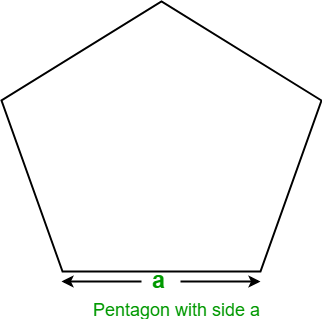# Program to find the Area of Pentagon

Given the side of a Pentagon, the task is to find the area of the Pentagon.

Examples:

Input : a = 5
Output: Area of Pentagon: 43.0119

Input : a = 10
Output: Area of Pentagon: 172.047745


A regular pentagon is a five sided geometric shape whose all sides and angles are equal. Its interior angles are of 108 degrees each and its exterior angles are 72 degrees each. The sum of interior angles of a pentagon is 540 degrees.## Recommended: Please try your approach on {IDE} first, before moving on to the solution.

Let a be the side of Pentagon, then the formula to find the area of Pentagon given by

Area =## C++

 // C++ program to find the area of Pentagon  #include  using namespace std;     // Function to find area of pentagon  float findArea(float a)  {      float area;           // Formula to find area      area = (sqrt(5 * (5 + 2 * (sqrt(5)))) * a * a) / 4;           return area;  }     // Driver code  int main()  {      float a = 5;             // function calling      cout << "Area of Pentagon: " << findArea(a);             return 0;  }

## Java

 // Java program to find the area of Pentagon  import java.io.*;     class GFG {             // Function to find area of pentagon      static float findArea(float a)      {          float area;                   // Formula to find area          area = (float)(Math.sqrt(5 * (5 + 2                  * (Math.sqrt(5)))) * a * a) / 4;                   return area;      }             // Driver code      public static void main (String[] args)       {          float a = 5;                     System.out.println("Area of Pentagon: "                                 + findArea(a));      }  }

## Python3

 # Python3 program to find   # the area of Pentagon     # Import Math module  # to use sqrt function  from math import sqrt     # Function to find   # area of pentagon     def findArea(a):             # Formula to find area      area = (sqrt(5 * (5 + 2 *            (sqrt(5)))) * a * a) / 4     return area            # Driver code  a = 5    # call function findArea()  # to calculate area of pentagon  # and print the calculated area  print("Area of Pentagon: ",                  findArea(a))      # This code is contributed   # by ihritik

## C#

 // C# program to find   // the area of Pentagon  using System;     class GFG   {             // Function to find       // area of pentagon      static float findArea(float a)      {          float area;                 // Formula to find area          area = (float)(Math.Sqrt(5 * (5 + 2 *                         (Math.Sqrt(5)))) *                                a * a) / 4;                 return area;      }             // Driver code      public static void Main ()       {          float a = 5;                     Console.WriteLine("Area of Pentagon: "+                                     findArea(a));      }  }     // This code is contributed   // by anuj_67.

## PHP

 

Output:

Area of Pentagon: 43.0119


Attention reader! Don’t stop learning now. Get hold of all the important DSA concepts with the DSA Self Paced Course at a student-friendly price and become industry ready.

My Personal Notes arrow_drop_upCheck out this Author's contributed articles.

If you like GeeksforGeeks and would like to contribute, you can also write an article using contribute.geeksforgeeks.org or mail your article to contribute@geeksforgeeks.org. See your article appearing on the GeeksforGeeks main page and help other Geeks.

Please Improve this article if you find anything incorrect by clicking on the "Improve Article" button below.

Improved By : vt_m, ihritik